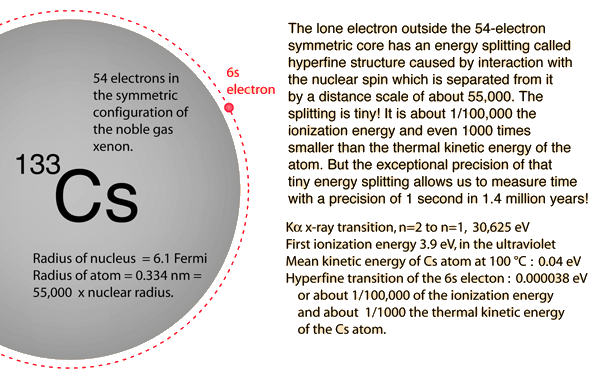Home » Posts tagged 'oscillation'

# Tag Archives: oscillation

## Is time always a measurement of movement of matter

Is the oscillation of energy levels of caesium in atomic clocks a form of motion (i. e. is time always a measurement of movement of matter)?

asked Gary Wederspahn

Answer:

The Oscillations in cesium clock is not mechanical. the radiation emission frequency between the two hyperfine levels of the atom is used as the standard for measuring time. Due to the fact that the standardisation of time is based on radiations, the atomic clocks are also called radio clocks.

Caesium clocks are the most accurate commercially produced time and frequency standards, and serve as the primary standard for the definition of the second in SI (the metric system). By definition, radiation produced by the transition between the two hyperfine ground states of caesium (in the absence of external influences such as the Earth’s magnetic field) has a frequency of exactly 9,192,631,770 Hz. That value was chosen so that the caesium second equalled, to the limit of human measuring ability in 1960 when it was adopted,

Very accurate clocks can be constructed by locking an electronic oscillator to the frequency of an atomic transition. The frequencies associated with such transitions are so reproducible that the definition of the second is now tied to the frequency associated with a transition in cesium-133:

1 second = 9,192, 631,770 cycles of the standard Cs-133 transitionCs Atomic clock

Ref http://hyperphysics.phy-astr.gsu.edu/hbase/acloc.html#c2

The oscillation in an atomic clock is between the nucleus of an atom and the surrounding electrons. This oscillation is not exactly a parallel to the balance wheel and hairspring of a clockwork watch, but the fact is that both use oscillations to keep track of passing time. The oscillation frequencies within the atom are determined by the mass of the nucleus and the gravity and electrostatic “spring” between the positive charge on the nucleus and the electron cloud surrounding it.## Effect on Time Period of a Simple Pendulum

“The bob of a simple pendulam is of iron.If a small magnet is placed just under the bob,then how the time period will be affected?”

Question Posted by Riya Mondal

The time period will decrease as the attraction of magnet increases the net downward pull (adding to g in the equation )

(It was a quick answer; Visitors are requested to respond)

## Equilibrium

Shubham Mukherjee asks:

“A ball suspended by a thread swings in a vertical plane so that its acceleration values in the extreme and lowest position are equal. Find the thread deflection angel in the extreme position?”

Answer will take some time. Visitors are requested to respond

## What is Simple Harmonic Motion?

Anil asked:

“What is Simple Harmonic Motion?”

Answer:

Please refer to the following Links for answer. (Please note that I prefer  the blunt questions arising out of natural curiosity)

http://en.wikipedia.org/wiki/Simple_harmonic_motion

http://www.splung.com/content/sid/2/page/shm

## Pendulum on Mount Everest !

Michelle Guzman Asked:

If you set a pendulum atop Mt. Everest, would the period be less than, greater than or equal to the period you would get in the lab?  Explain.

Ans:

As you know, the acceleration due to gravity decreases as we go upwards from surface of earth.

The time period of a simple pendulum is given byWhere l is the length of the pendulum, and g is the acceleration due to gravity.

From the equation, we can conclude that, the Time period of the pendulum increases (i.e. it goes slower)  if the pendulum is set up on top of Mount Everest.

For Creative and Critical Thinkers

Here we have not considered the change in temperature between the laboratory conditions and the temperature on top of Mount Everest which is very low. A decrease in temperature would decrease the length of the pendulum (Unless it is made up of such a substance which does not change its length with change in  temperature) and will also decrease the time period of the pendulum, making it move faster.

My first answer is correct only if the laboratory conditions are the same as on top of Mt. Everest or vice versa, except for the difference in altitude (height)

Ask Physics

### Visitors So Far @ AskPhysics

• 2,116,393 hits

### Subscribe to Blog via Email

Enter your email address to subscribe to this blog and receive notifications of new posts by email.

Join 4,004 other subscribers

### askphysics@TwitterAsk Physics
@askphysics